# TidyTutorial 3: Plotting with ggplot (and writing a function!)

In this tutorial we will load in the data created in the second tutorial and make some plots

Plotting in Tidyvserse is handled by the ggplot package

library

``library(tidyverse)``

###Read in the data we created in TidyTutorial 2

``dat<-read.csv("Tidy2_dat.csv")``

###This data contains five variables: id, group, time, score, and score_2

###We will use this data to go over the basics of plotting with ggplot

``head(dat)``
``````##   id group time score score_2
## 1  1     0    0  0.30    0.30
## 2  1     0    1  0.26    0.76
## 3  1     0    2  0.53    1.53
## 4  1     0    3  1.90    3.40
## 5  1     0    4 -1.77    0.23
## 6  1     0    5 -3.61   -1.11``````

#Tidyverse part 1: ggplot (it’s for plotting)

##We can “initiate” a plot with the ggplot function

``dat %>% ggplot()``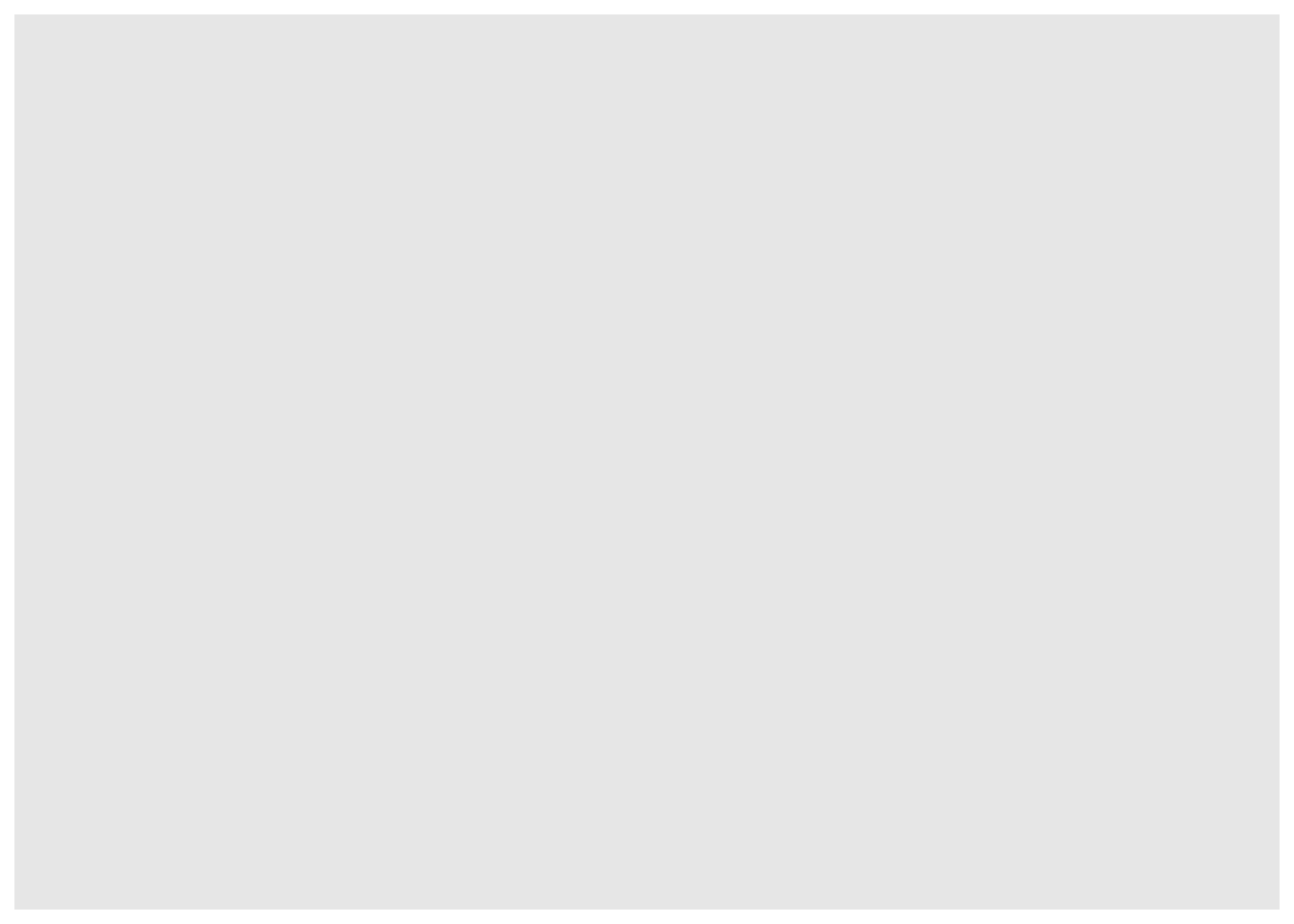``#Here we have a "blank" plot``

##We can then add a some ‘aesthetics’ with aes

``dat %>% ggplot(aes(x=time, y=score))``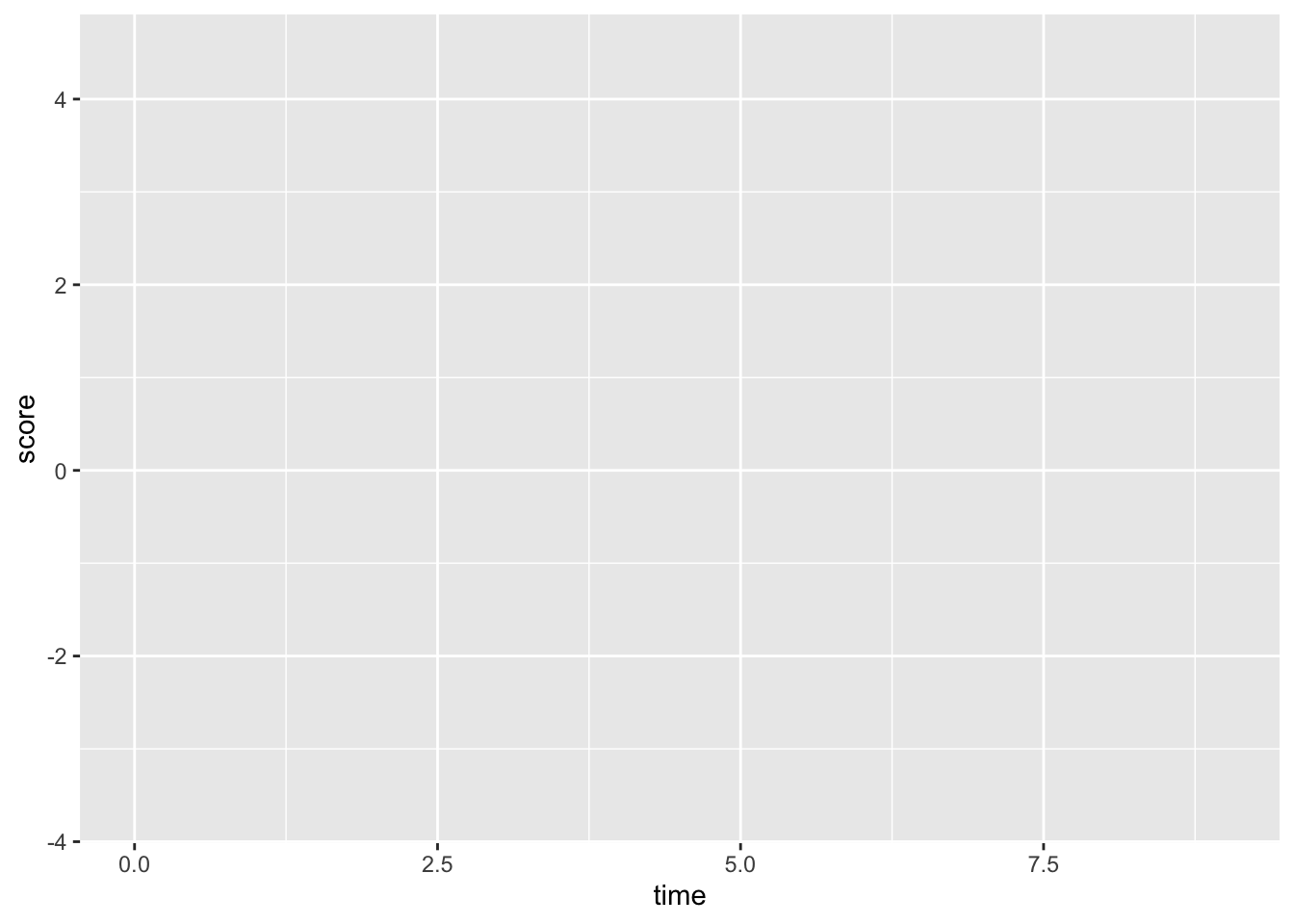``#This sets the x and y axis, but we still have not added any data to the plot``

##Now we can add a ‘layer’ to the plot using ‘+ geom’

``dat %>% ggplot(aes(x=time, y=score))+geom_point()``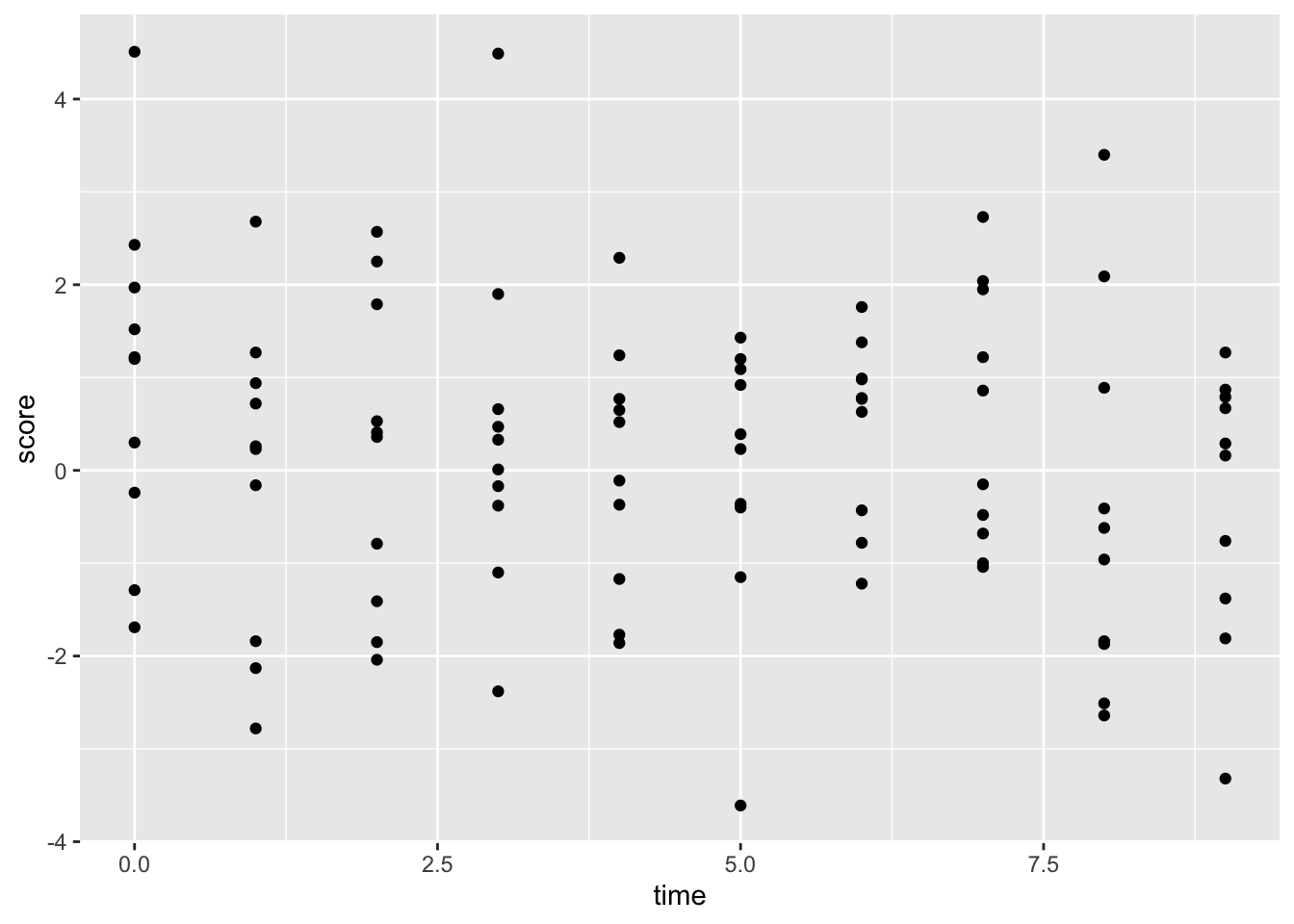``#This is a scatter plot showing the time series of the "score" variable ``

##Here we can try and connect the dots using “geom_line”

``dat %>% ggplot(aes(x=time, y=score))+geom_point()+geom_line()``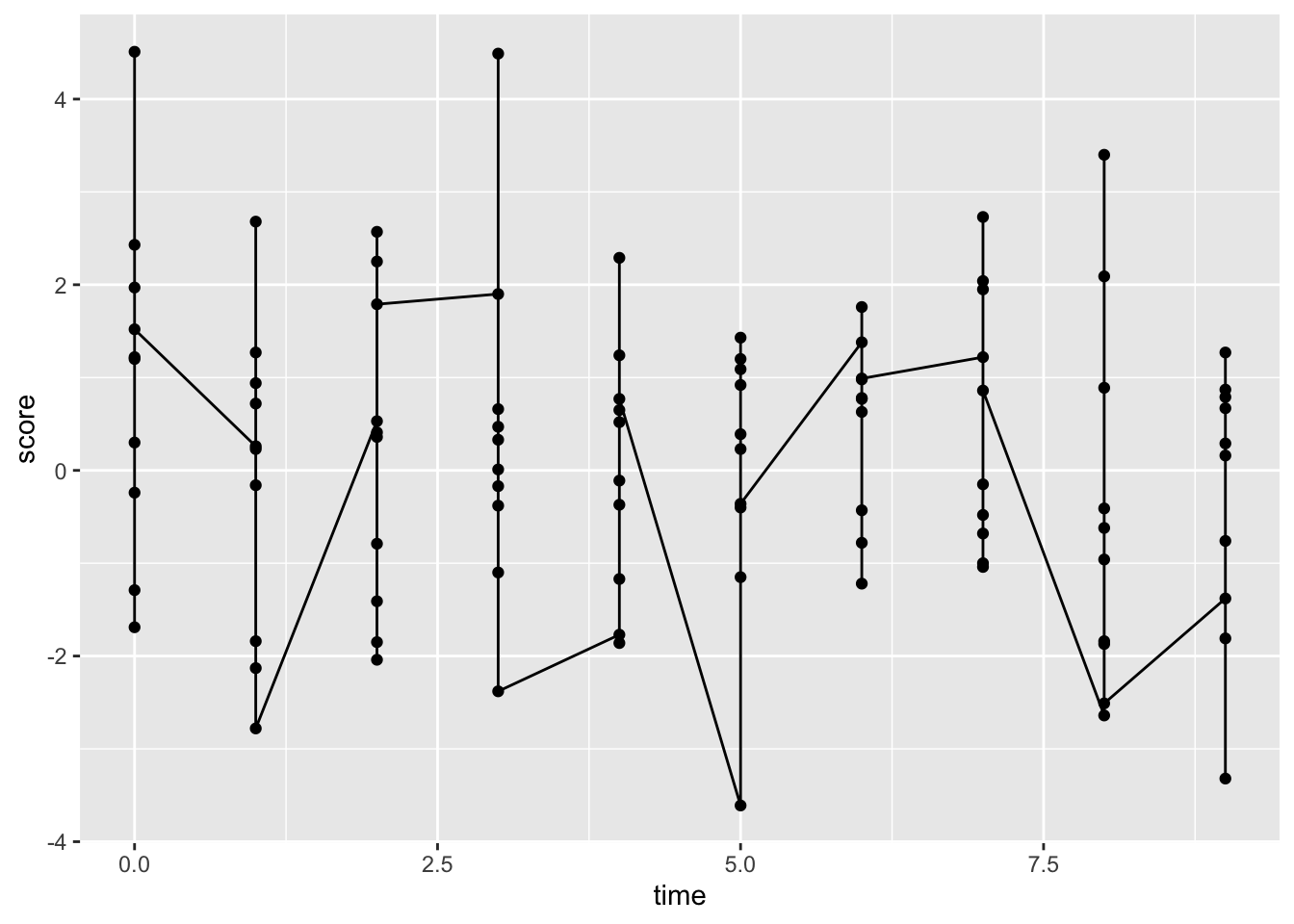###Hmm….that seems wrong

##To get what we want we need to add a ‘group’ variable

``````dat %>%
ggplot(aes(x=time, y=score, group=id))+ #set group to id
geom_point()+
geom_line()``````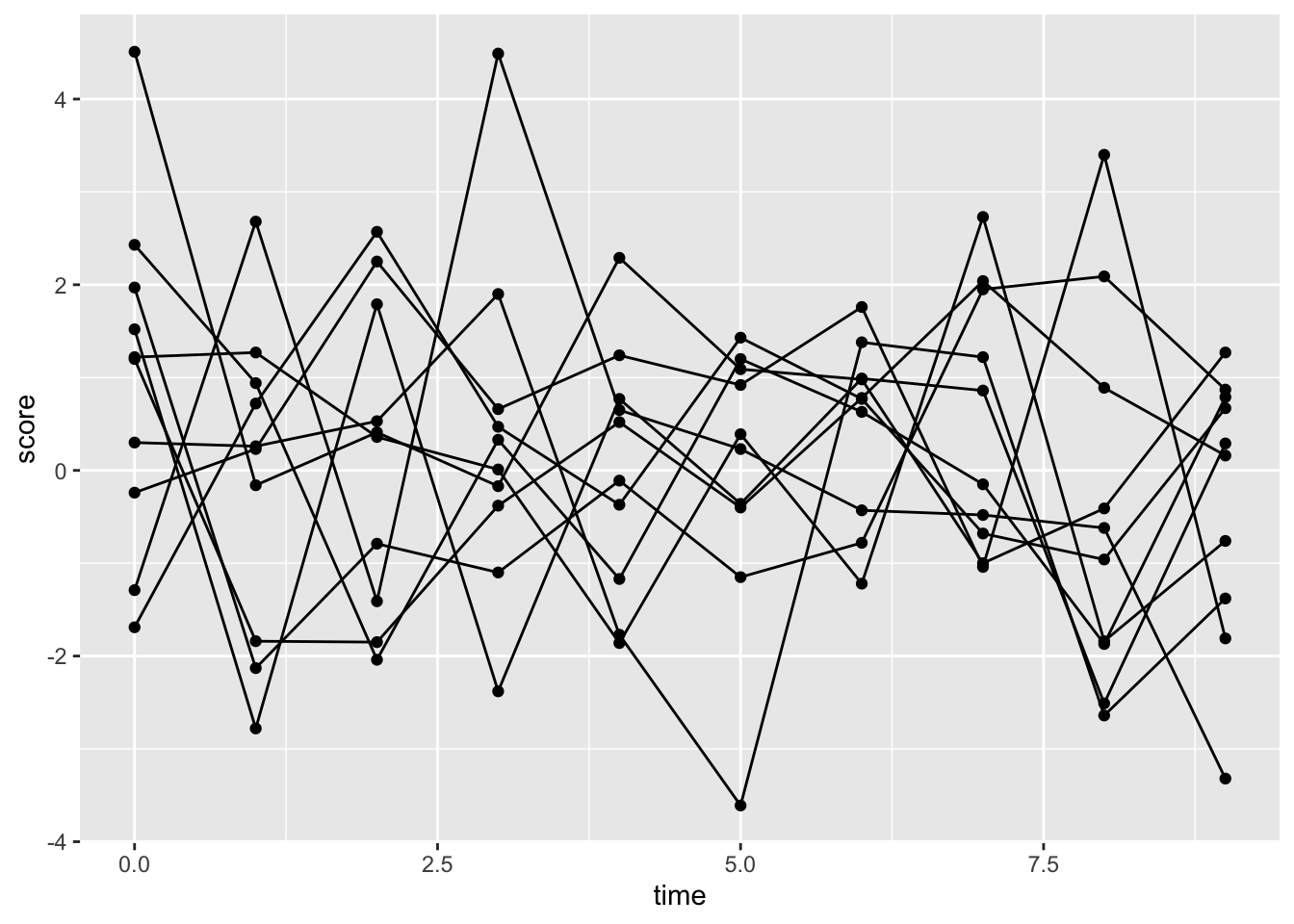``#Person-specific time-series of scores``

##We can set the lines to have a different color for each ID. This will automatically add a legend

``````dat %>%
ggplot(aes(x=time, y=score, group=id, color=factor(id)))+geom_point()+geom_line()``````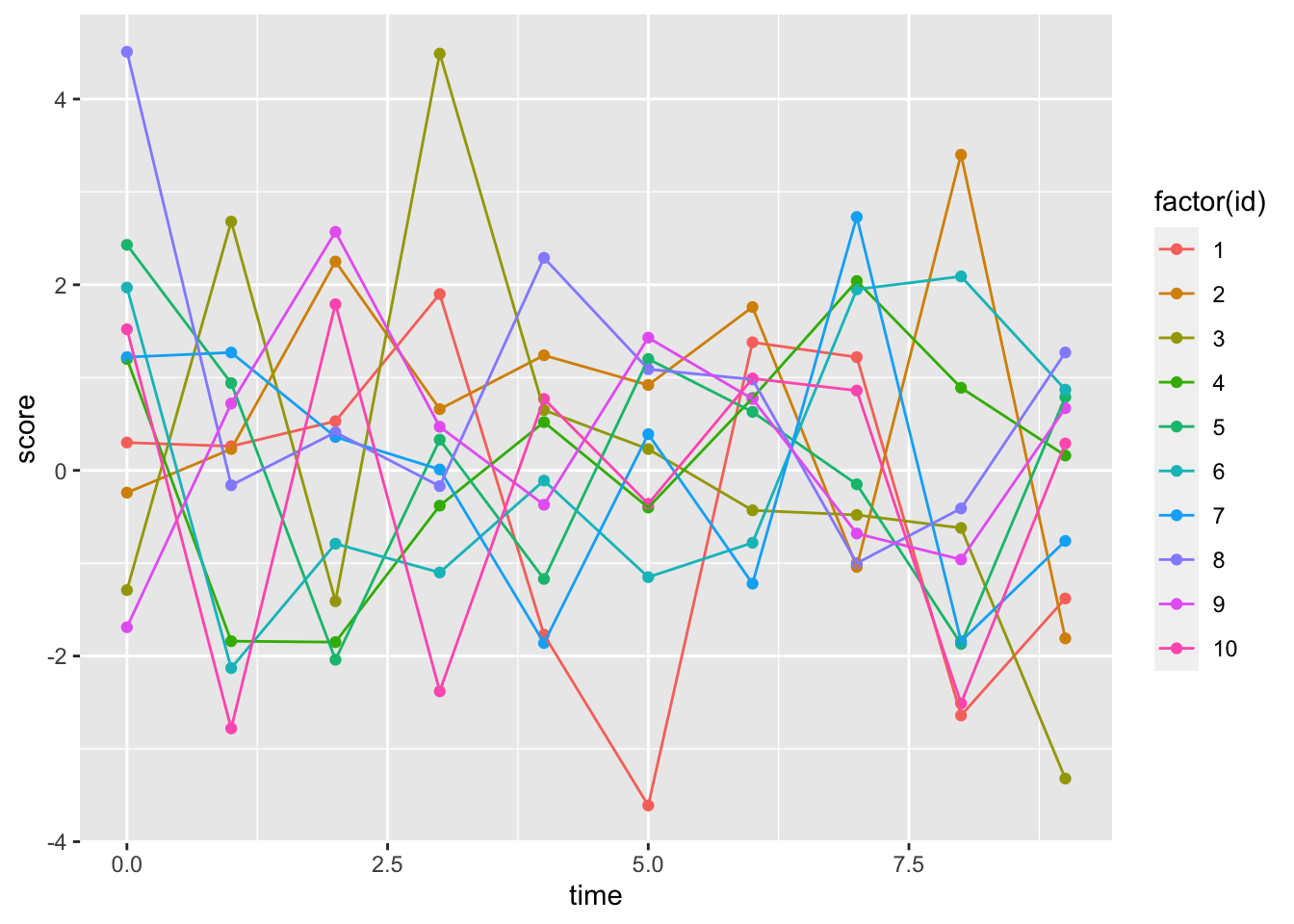``#Person-specific time-series``

##We can also modify the ‘theme’ of the plot; themes change how a plot looks

``````dat %>%
ggplot(aes(x=time, y=score, group=id, color=factor(id)))+
geom_point()+
geom_line()+
theme_bw() #This is may favorite theme, there are also lots of others ``````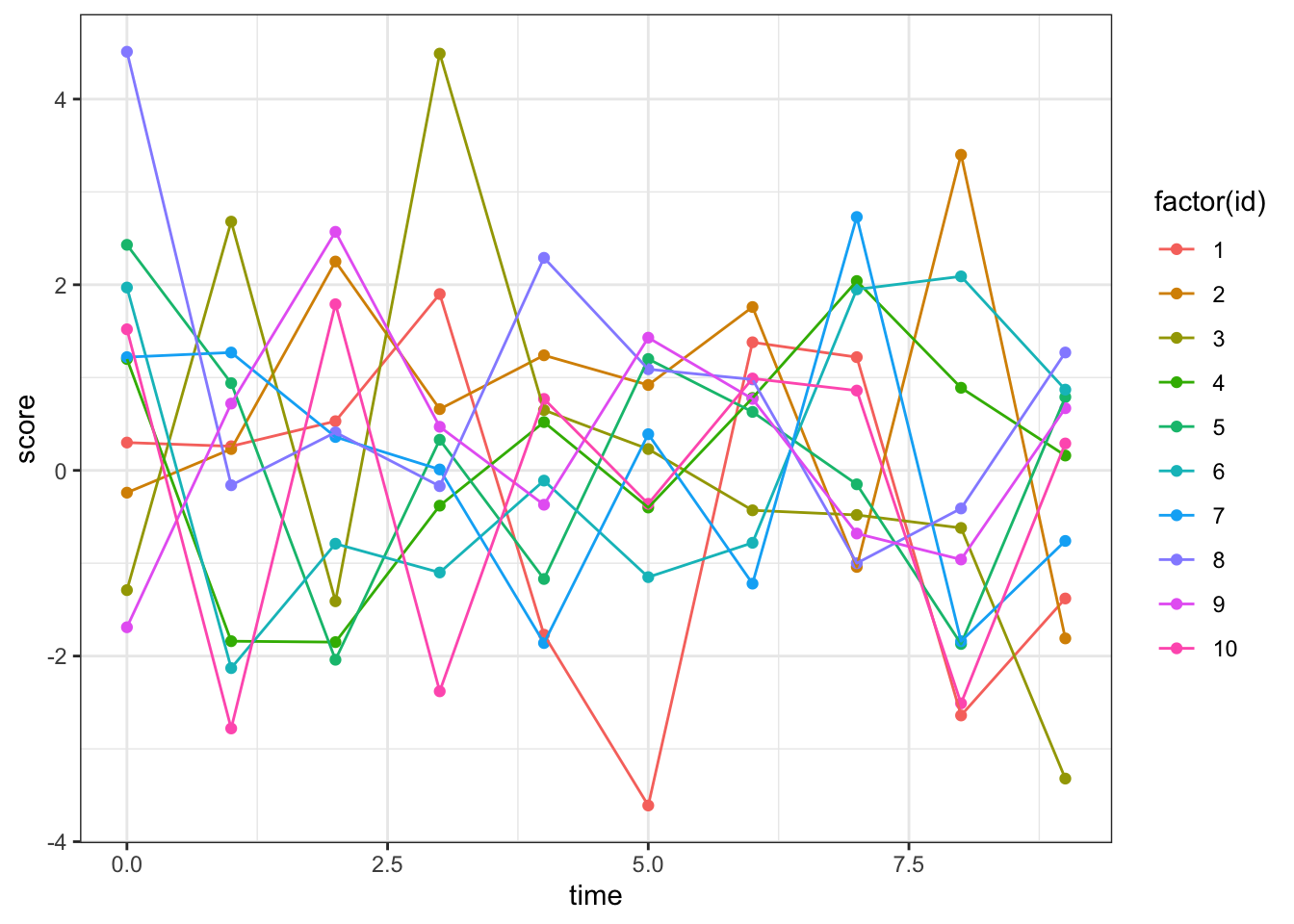``#Person-specific time-series with black+white theme``

##Let’s get rid of the legend on the right side of the plot

``````dat %>%
ggplot(aes(x=time, y=score, group=id, color=factor(id)))+
geom_point()+
geom_line()+
theme_bw()+
theme(legend.position = "none") #This makes legends go away``````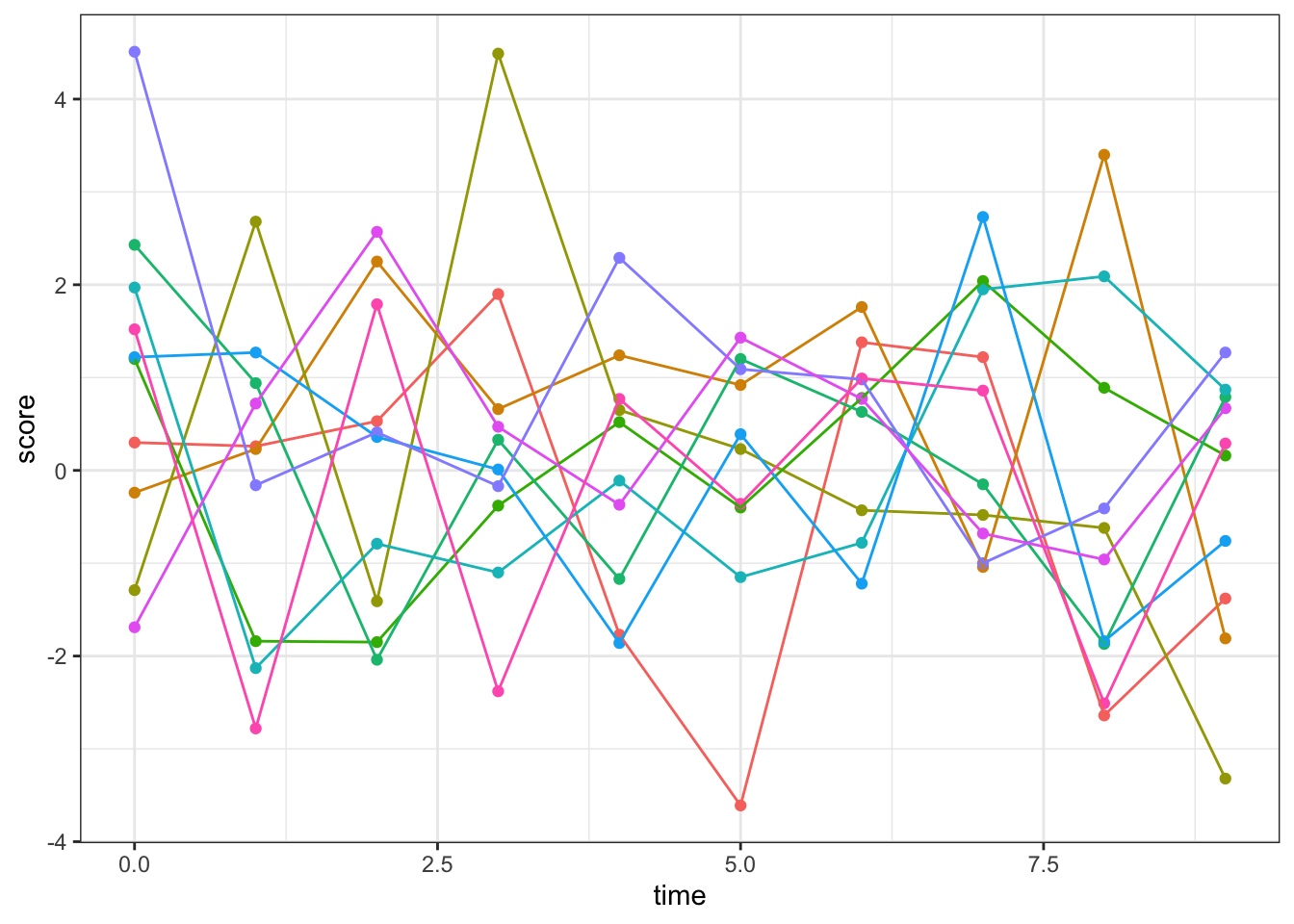``#Person-specific time-series with black+white theme and no legend``

##Now let’s produce the same plot for score 2

``````dat %>%
ggplot(aes(x=time, y=score_2, group=id, color=factor(id)))+ #note the change in the 'y=' input
geom_point()+
geom_line()+
theme_bw()+
theme(legend.position = "none")````````#Person-specific time-series with black+white theme and no legend``

All of these seem to increase in a linear fashion (which we set them to do in the previous tutorial)

##Now we can add a regression line

``````dat %>%
ggplot(aes(x=time, y=score_2))+ #note the change in the 'y=' input
geom_point()+
geom_smooth(method = "lm")+ #This adds a linear regression line
geom_line(aes(group=factor(id), color=factor(id)), alpha=0.75)+
theme_bw()+
theme(legend.position = "none")``````
``## `geom_smooth()` using formula 'y ~ x'``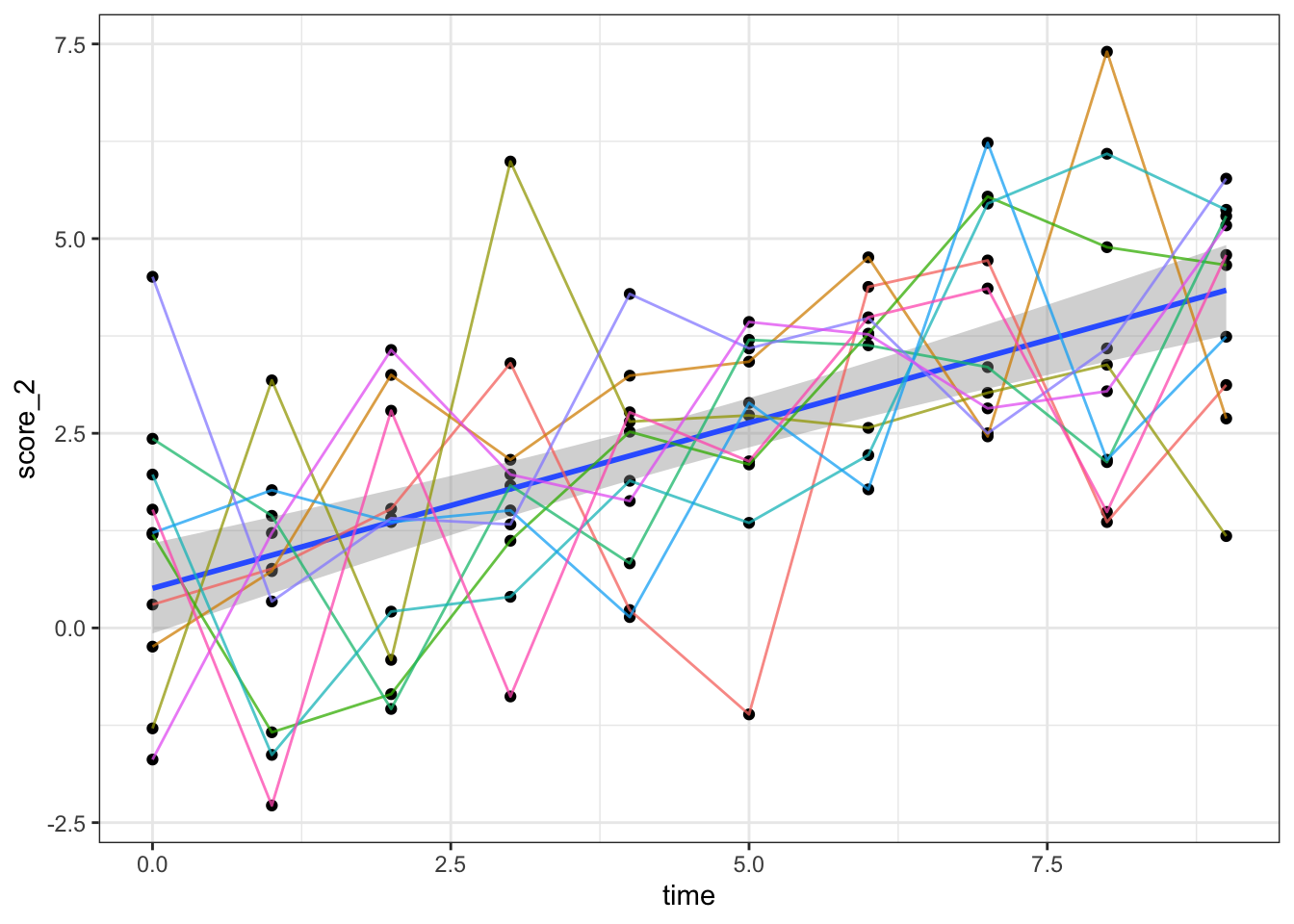``#Person-specific time-series with black+white theme and no legend``

##We can add a linear model (it’s in base R- tidy options are available but I use base for lm)

``````dat %>%
ggplot(aes(x=time, y=score_2))+ #note the change in the 'y=' input
geom_point()+
geom_smooth(method = "lm")+ #This adds a linear regression line
geom_line(aes(group=factor(id), color=factor(id)), alpha=0.75)+
theme_bw()+
theme(legend.position = "none")``````
``## `geom_smooth()` using formula 'y ~ x'````dat %>% lm(score_2 ~ time, data=.) %>% summary()``
``````##
## Call:
## lm(formula = score_2 ~ time, data = .)
##
## Residuals:
##     Min      1Q  Median      3Q     Max
## -3.7459 -0.9966  0.0721  0.9601  4.2048
##
## Coefficients:
##             Estimate Std. Error t value Pr(>|t|)
## (Intercept)  0.50915    0.29216   1.743   0.0845 .
## time         0.42535    0.05473   7.772 7.75e-12 ***
## ---
## Signif. codes:  0 '***' 0.001 '**' 0.01 '*' 0.05 '.' 0.1 ' ' 1
##
## Residual standard error: 1.572 on 98 degrees of freedom
## Multiple R-squared:  0.3813, Adjusted R-squared:  0.375
## F-statistic: 60.41 on 1 and 98 DF,  p-value: 7.745e-12``````

This regression model shows that there is a significant increase in score 2 over time, on average

##We could also plot the distributions of score across all time point for each ID

``````dat %>%
ggplot(aes(x=score_2))+ #note the change in the 'y=' input
geom_density(aes(group=factor(id), fill=factor(id)), alpha=0.8)+
theme_bw()+
theme(legend.position = "none")+
facet_wrap(~id, ncol = 5)``````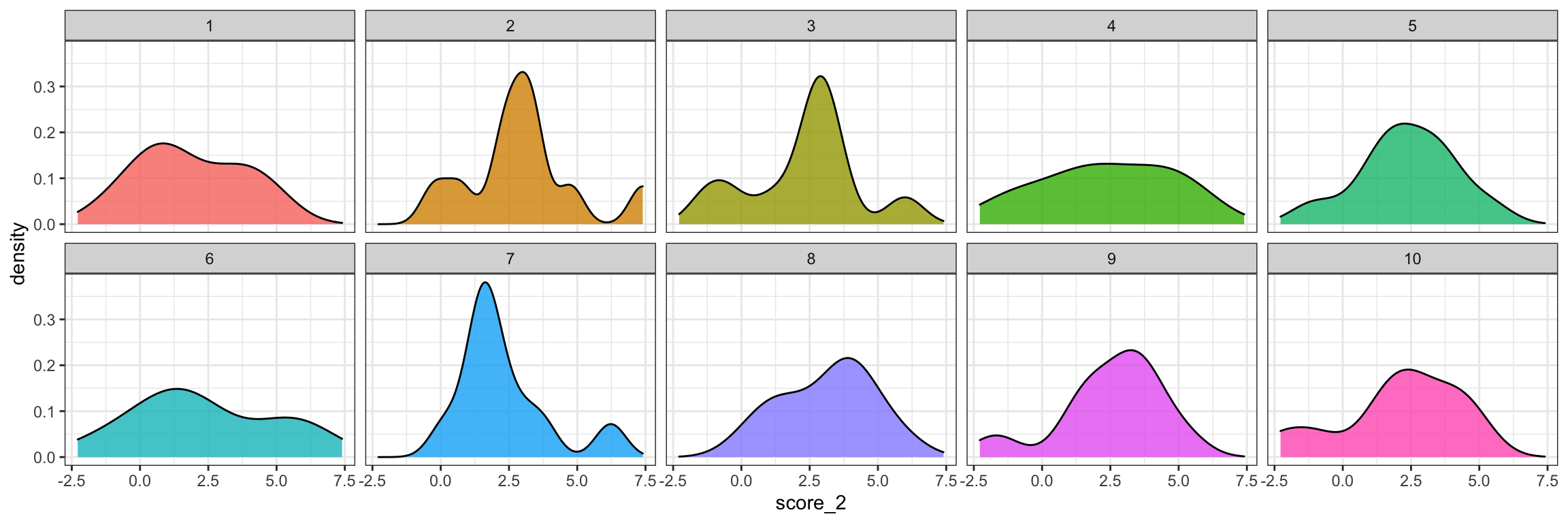##We could also plot the bivariate relationship between ‘score’ and ‘score_2’

``````dat %>%
ggplot(aes(x=score, y=score_2))+
geom_point(aes(group=factor(id), color=factor(id)))+
theme_bw()+
theme(legend.position = "none")``````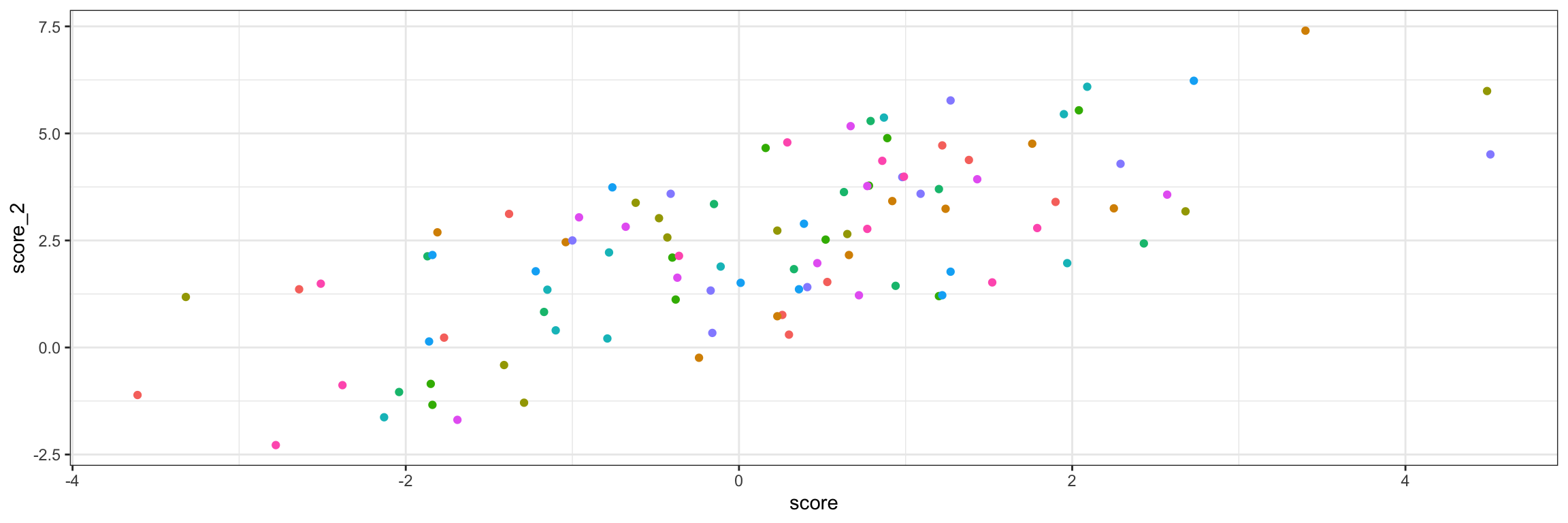##Now we can write our first function. Functions are great if you plan to make the same kind of plot a bunch of times

``````bivariate_plot_function<-function(x){x %>% ggplot(aes(x=score, y=score_2))+
geom_point(aes(group=factor(id), color=factor(id)))+
theme_bw()+
theme(legend.position = "none")}``````

##Let’s apply this function to to our data set

``bivariate_plot_function(dat)``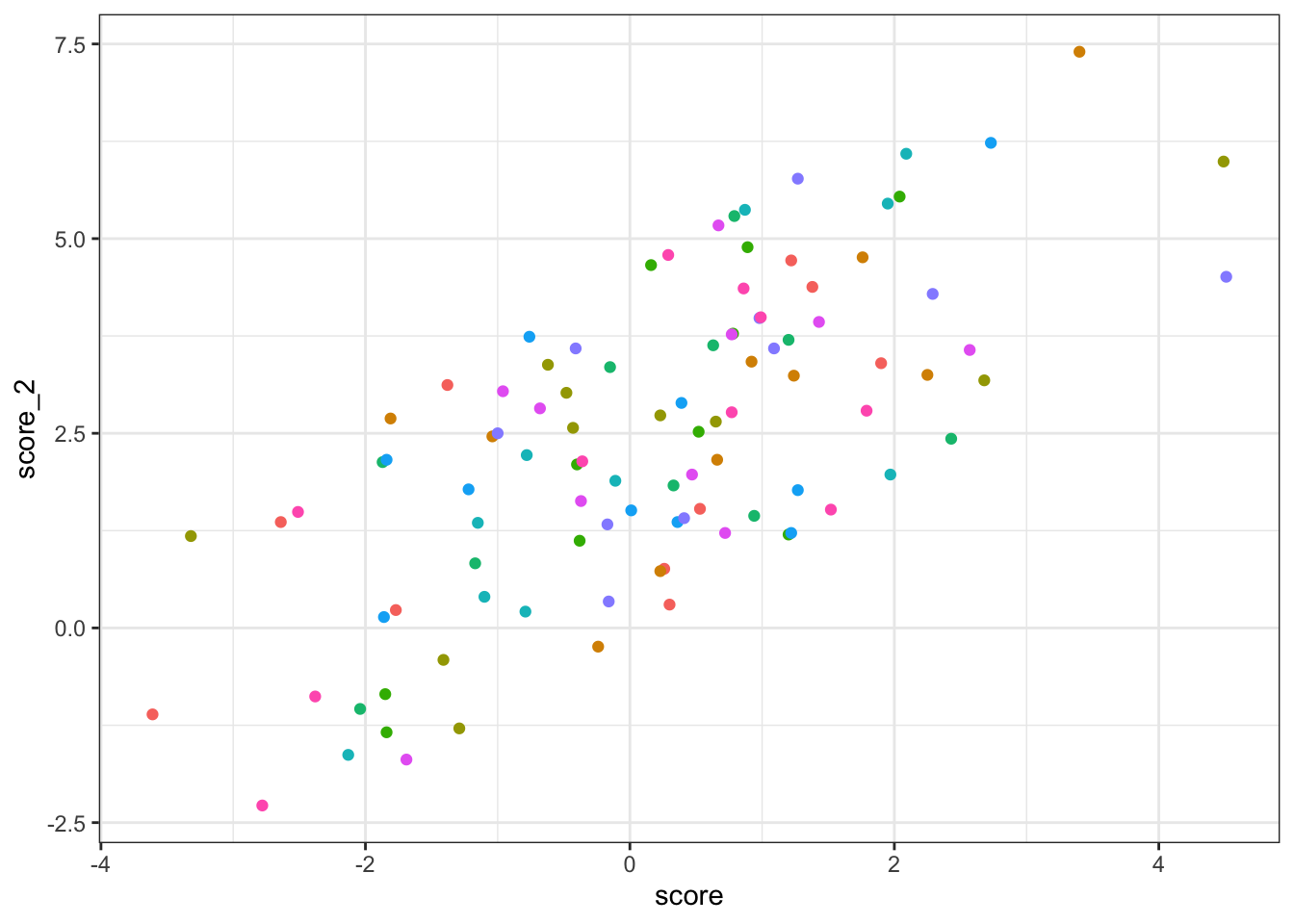##Alright, no data to write out for tutorial 3. But, what if we wanted to save one of these plots?

##To save a plot, first assign it to an object and then use ‘ggsave’

``````plot_1<-dat %>%
ggplot(aes(x=time, y=score_2))+ #note the change in the 'y=' input
geom_point()+
geom_smooth(method = "lm")+ #This adds a linear regression line
geom_line(aes(group=factor(id), color=factor(id)), alpha=0.75)+
theme_bw()+
theme(legend.position = "none")

#Here is our person-specific time-series plot with a regression line``````

##Let’s view the plot object we just assigned

``plot_1``
``## `geom_smooth()` using formula 'y ~ x'``##Alright, now we can save it using ggsave. This will be saved as a pdf but you can alos use .png or other formats

``ggsave(plot_1, file="plot_1.pdf", width = 12, height = 8)``
``## `geom_smooth()` using formula 'y ~ x'``

###Plots are useful for looking at trends in your data. Modeling these trends statistically will be covered in tutorial #4